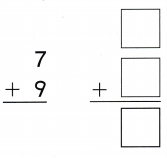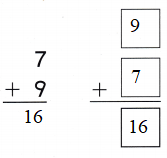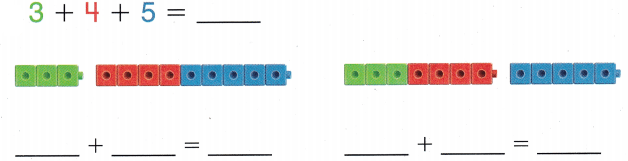Refer to our Texas Go Math Grade 1 Answer Key Pdf to score good marks in the exams. Test yourself by practicing the problems from Texas Go Math Grade 1 Module 12 Assessment Answer Key.

Concepts and Skills

Question 1.Question 2.Look at the. Complete the addition sentences showing two ways to find the sum. (TEKS 1.5.F, 1.5.G)

Question 3.Answer: 3 + 4 + 5 = 12

Question 4.
Write the number sentence.
Maria has 3 cats. Jim has 2 cats. Cheryl has 5 cats. How many cats do they have? (TEKS 1.5.F, 1.5.G )__________ + __________ + __________ = __________ cats
Answer: 3 + 2 + 5 = 10 cats

Question 5.
Pete sends 4 letters. Then he sends 3 more letters. Then he sends 2 more letters. How many letters did Pete send? (TEKS 1.5.F, 1.S.G)(A) 7
(B) 6
(C) 9
Given,
Pete sends 4 letters.
Then he sends 3 more letters.
Then he sends 2 more letters.
4 + 3 + 2 = 9
Thus the correct answer is option C.

Question 6.
Which shows the same addends in a different order? (TEKS 1.5.F)
7 + 6 = 13
(A) 8 + 6 = 14
(B) 6 + 7 = 13
(C) 9 + 6 = 15
6 + 7 = 13
Thus the correct answer is option C.

Question 7.
What is the sum of 7 + 7 + 3? (TEKS 1.5.F, 1.5.G)
(A) 17
(B) 10
(C) 14